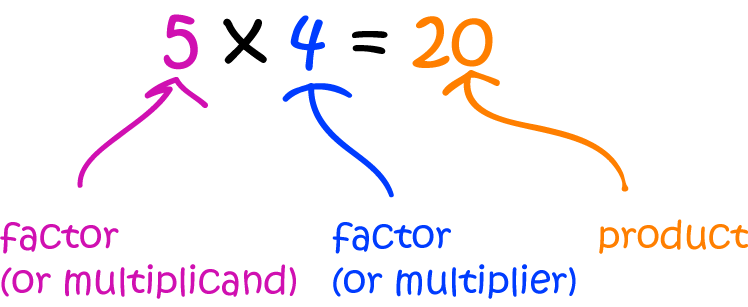# Definition of MultiplierA multiplier is the number that does the multiplying in a multiplication sum.

The number that gets multiplied is called the "multiplicand".

However, these terms are confusing because you can perform a multiplication in any order you like. So, the term "factor" is more commonly used to refer to both the "multiplicand" and the "multiplier".

### Description

The aim of this dictionary is to provide definitions to common mathematical terms. Students learn a new math skill every week at school, sometimes just before they start a new skill, if they want to look at what a specific term means, this is where this dictionary will become handy and a go-to guide for a student.

### Audience

Year 1 to Year 12 students

### Learning Objectives

Learn common math terms starting with letter M

Author: Subject Coach
You must be logged in as Student to ask a Question.